# WBBSE Class 9 Physical Science Notes Chapter 2 Force and Motion

Comprehensive WBBSE Class 9 Physical Science Notes Chapter 2 Force and Motion can help students make connections between concepts.

## Force and Motion Class 9 WBBSE Notes

Rest : If a body does not change its position with respect to time, the body is said to be at rest and that body is called static body.

Motion : If a body changes its position with respect to time, the body is said to be in motion and that body is called kinetic body.

Rest and motion is always a relative term : An object in one situation may be at rest, while in another situation the same object may appear to be in motion.

For example, a man sitting in a running train finds a man standing on the platform or in a field or a tree or a building all moving backwards, i.e. in motion. But actually, to the man on the field, the tree, the building etc. appear to be stationary, while the man in the train is moving forward.

Classification of motion :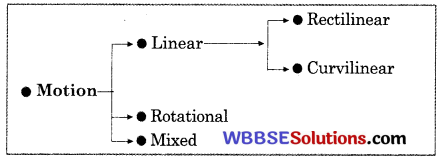Linear motion : If any particle moves along any line, then the motion of that particle is called linear motion.

Straight linear motion : If any particle moves along any straight line and any particle of the body travels the same distance at same speed, then the motion is called straight linear motion.

Curve linear motion : If any particle does not move in a definite direction but moves in curvilinear way and changes its direction in that way, then the motion is called curve linear motion.

Circular motion : If any particle moves around the particular point or axis, then this motion is called circular motion.
Example: The motion of electric fan.

Mixed motion : If any motion is a combined form of linear motion and circular motion, then that motion is called mixed motion.

Example: The moving wheel car. Here the circular motion of wheel produces linear motion.
Concept of a particle: A particle is a geometrical mass point or a material body of neglígible dimensions.

Reference body : For determining whether a body is at rest or in motion we must compare the motion of the body with some other body, called the reference body.

Reference frame : To locate the position of body with respect to the reference body, a system of co-ordinates fixed to the reference body is constructed. This is called reference frame.Types of reference frames :

Inertial frame of reference : A frame of reference which is either at rest or moving with uniform velocity is called an inertial frame of reference.
In this frame of reference, Newton’s first law of motion is valid.

Example :

• A frame of reference remaining fixed with respect to stars is an inertial frame of reference.
• A space ship moving in space without spinning and with its engine shut off is an inertial frame of reference.
• A train moving on a horizontal track with constant velocity is an inertial frame of reference.

Non-inertial frame of reference : A frame of reference which is accelerated is called non-inertial frame of reference.
In this frame of reference, Newton’s first law of motion is not valid.
Example : A ball is lying on the floor of a stationary bus. When the bus is accelerated or retarded, the ball starts moving. The bus is said to an example of non-inertial or accelerated frame of reference.

Motions in one, two or three dimensions :

One dimensional motion : If the motion of a particle be along a straight line, the motion is one dimensional.
Example :

• A train moving along a straight track.
• A body falling freely under gravity.

Two dimensional motion : If the motion of a particle be in a plane, i.e. when two of the three co-ordinates are required to specify the position of the particle in motion, the motion is called two dimensional.

Example :

• The earth revolving round the sun.
• An insect crawling on the floor.Three dimensional motion : If the motion of a particle be in a space, i.e. when all the three co-ordinates are required to specify the position of the particle in motion, the motion is called three dimensional.

Example :

• A flying bird
• A flying aeroplane
• A flying kite

Position of a particle moving along a straight line : The position of a particle moving along a straight line is described by a quantity x. The numerical value of x, together with the unit, gives the distance of the particle from the origin, and the sign of x denotes whether the particle is in the positive direction or in the negative direction.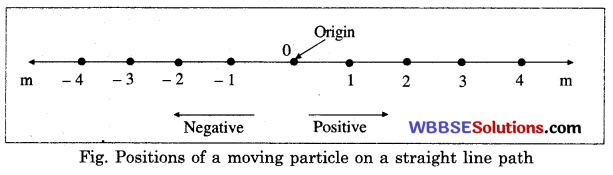Fig. Positions of a moving particle on a straight line path
To describe the position of the particle at a given time, we have to specify :

• its distance from the origin
• whether it is in the positive direction or in the negative direction as seen from the origin.

Distance traversed : The length of the actual path traversed by a particle during motion in a given interval of time is called distance traversed by the particle.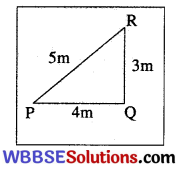Suppose a man goes from P to Q, a distance of 4 m. Then he moves a distance QR of length 3 m at right angle to PQ.

Thus starting from P, he reaches finally at R travelling a total distance PQ + QR = (4 + 3) m = 7 m.
Unit (SI) of distance traversed : meter (m).
Distance traversed : Scalar quantity

Displacement : The displacement of a moving particle in a given interval of time is the shortest distance between the two positions of the moving particle in a particular direction and is given by the vector drawn from the initial position of the particle to its final position.

Units of displacement :

• CGS : cm
• SI : mDimensions of displacement : [M0 L1 T0]

The characteristics of displacement are :

1. Displacement has the unit of length.
2. The displacement may be positive, negative or zero.
3. The magnitude of the displacement of a moving particle between two points gives the shortest distance between them.
4. The displacement of a moving particle between two points does not give us any idea about the path followed by the moving particle.
5. The displacement of a moving particle between two points has a unique value.
6. The actual distance travelled by the moving particle may be greater than or equal to the magnitude of the displacement.
7. The displacement remains unaltered, even if the origin of the co-ordinate axis be shifted.
8. As a particle cannot be at two different positions at the same time, so the displacement is a single valued function of time.
9. The displacement changes if its direction be changed.

Diffference between displacement and distance

 Displacement Distance i. The distance moved by a particle in any particular direction is called the displacement of the particle. i. Total path covered by a particle in a straight or curved path is called distance traversed by the particle. ii. It is a vector quantity. ii. It is a scalar quantity.

Speed : Rate of change of position of a body with respect to time is known as its speed.
It is measured by the distance covered by body in unit time.Characteristics of speed :

1. Speed does not give any idea about the direction of motion.
2. Speed can be positive, but never negative or zero.
3. Speed is a scalar quantity.Units of speed :

• CGS: cmsM1
• SI: ms-1

The dimensions of speed are : M0 L1 T-1]

Types of Speed :

Uniform speed : The speed of a body is said to be uniform, if it covers equal distance in equal intervals of time, however small these intervals may be.

Non-uniform speed or variable speed : If an object does not cover equal distances in equal time-intervals, its speed is called non-uniform or variable speed.

Average speed: When a body is moving with variable speed, the constant speed with which the body covers the same distance in a given time as it does while moving with variable speed during the given time, is called the average speed.If a body moves distances S1, S2, S3, …. etc. in times t1, t2, t3 …. etc. respectively, then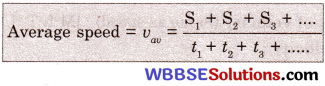Instantaneous speed: The instantaneous speed at a point is defined as the limit of the average speed over a path length that tends to become zero but always includes that point.
Thus, if the moving particle covers a path △S in time interval △t, then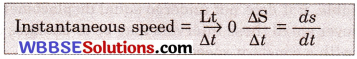Whenever we mention about the speed of a particle, it always means the instantaneous speed, if not mentioned otherwise. In case of uniform motion of a particle, the instantaneous speed is equal to the uniform speed.Typical speeds of some objects :

 Average speed of a tortoise 05 km/h Average walking speed of man 6 km/h Maximum speed of a bee 16 km/h Maximum speed of a cheetah 96 km/h Maximum speed of a falcon 152 km/h Maximum speed of a typical fast train 225 km/h Wind speed in a light breeze 32 km/h Wind speed in a hurricane 320 km/h The speed of the earth moving round the sun 1.072 × 105 km/h

If not mentioned otherwise, the term velocity means instantaneous velocity.

Difference between speed and velocity :

 Speed Velocity i. Speed is the time rate of change of position of a body in any direction. i. Velocity of a body is the time rate of change of its displacement. ii. Speed is a scalar quantity, i.e. it has only magnitude but no definite direction. ii. Velocity is a vector quantity, i.e. it has both magnitude and also a particular direction. iii. Instantaneous speed is the magnitude of instantaneous velocity. iii. With a proper direction attached to the instantaneous speed, instantaneous velocity is obtained. iv. A body moving with uniform speed may not have uniform velocity. iv. A body moving with uniform velocity always possesses uniform speed. v. Speed can be positive but never negative or zero. v. Velocity can be positive, negative or zero.

Acceleration : The rate of increase of velocity of a body with respect to time is called its acceleration.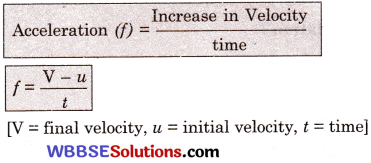Characteristics of velocity :

1. When the velocity increases, the acceleration is called positive and when it decreases, it is called negative or retardation or deceleration.
2. Acceleration has both magnitude and direction, so it is a vector quantity.

Units of acceleration :

• CGS: cms2
• SI: ms2

Dimensions of acceleration : [M0 L1 T2]

Types of acceleration :

Uniform acceleration : The acceleration of a moving body is said to be uniform if its velocity changes be equal amounts in equal intervals of time, however small the intervals may be.

Non-uniform or variable acceleration : The acceleration of a moving body is said to be variable, if it does not have equal changes in velocity in equal intervals of time, however small these intervals may be.

Average acceleration : When a body is moving with variable acceleration, then the average acceleration of the body is defined as the ratio of the total change in velocity of the body to the total time taken.

Instantaneous acceleration : The instantaneous acceleration of a particle at a point at a certain instant of time is the limit of the average acceleration over a displacement that tends to be zero, but always includes the given point.

Thus, if the moving particle undergoes a change of velocity of $$\overrightarrow{\Delta \mathrm{V}}$$ in time interval Δt, thenIf not mentioned otherwise, the term acceleration means instantaneous acceleration.
Retardation : The rate of decrease of velocity of a body with respect to time is called retardation.

Characteristics of retardation :

1. Retardation is opposite to the acceleration and thus it is considered as negative acceleration.
2. Retardation has both magnitude and direction, and hence it is vector quantity.

Units of retardation :

• CGS : cms2
• SI: ms2

Dimensions of retardation : [M0L1T2]

Types of retardation :

1. Uniform retardation : The retardation of a moving body is said to be uniform if its velocity changes by equal amounts in equal intervals of time, however small the intervals may be.
2. Non-uniform retardation: The retardation of a moving body is said to be variable, if it does not have equal changes in velocity in equal intervals of time.
3. Instantaneous retardation : The instantaneous retardation of a particle at a point at a certain instant of time is the limit of average retardation over a displacement that tends to be zero but always includes the given point.

Graphical Representation of Motion : A graph is a very powerful method of representing information.

To describe the motion of an object, we can use line graphs. In this case, line graphs show dependence of one such as distance or velocity, on another quantity, such as time.Plotting a graph : A graph is plotted to display the relation between two quantities. Generally, one of the two quantities changes independently and the other quantity depends on it.

Choosing the axes: Draw two perpendicular lines, crossing each other at a point. Each line represents one of the two quantities to be plotted. Generally, a horizontal line from left to right is drawn to represent the independent quantity, and a perpendicular line is drawn to represent the dependent quantity. These lines are called the x-axis and the y-axis, or the horizontal axis and the vertical axis respectively.

Choosing the scale : The size of the paper on which a graph is drawn is limited. On the available length of the axes, values are marked at equal distances. This is done in such a way that all the values of the quantity represented on the axis can be accommodated in the available length.

Plotting the points : Each set of values of the two quantities is represented by a point on the graph.

Joining the points: Once all the points corresponding to the available sets of values of the quantities are plotted, they are joined by a smooth curve to get the graph.

Velocity-time graph : Velocity-time graphs under different conditions are shown in the Fig. 1 to Fig. 4. In Fig. 1, velocity-time graph for a particle moving with uniform velocity is shown.As the velocity V is uniform, so the plot of the velocity against time would be a straight line parallel to the time axis. The distance moved in time t is equal to v t, which is seen from the graph as the area of the rectangle OABC. Thus the distance travelled by a particle in uniform motion is given by the area under the velocity-time graph.

In Fig. 2, velocity-time graph for a particle moving in straight line with uniform acceleration and starting with initial velocity u = 0 is shown. Same motion starting with initial velocity u is shown in Fig.3. In both the cases velocity-time curves are straight line. In each case the constant slope of the straight line gives the uniform acceleration.

In Fig. 4, velocity-time graph for a particle moving with uniform retardation in a straight line is shown. The curve is again a straight line but the slope is negative. In all the cases, the distance moved by the particle is given by the area under the velocity-time graph.Position-time graph :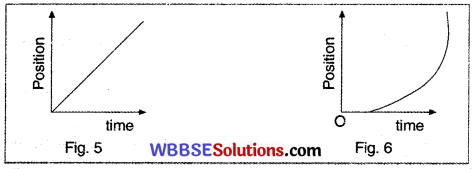In Fig. 5 position-time graph of a particle moving with uniform velocity is shown. It is a straight line and the slope of the curve gives the uniform velocity of the particle.

In Fig. 6. position-time graph for a particle moving with uniform acceleration in a straight line is given. The graph is parabolic in nature. Here the slope of the curve gives the average velocity over certain interval of time.
Equations for motion with uniform acceleration: Consider a particle
moving with acceleration ‘a’ along a straight line, starting with initial velocity ‘u’ attains a velocity ‘V’ in interval of time ‘t’ and traverses a distance ‘S’ in this interval.

In Fig. 7, the velocity-time graph for the motion is shown. Here OD = u and AC = v and OA = t.
From the graph we haveAC = AB+BC
or, v = u + a t

Again the distance ‘S’ covered by the particle in the time interval ‘t’ is given by the area of the trapezium OACD.

or, s = area of the trapezium OACD
= area of the rectangle OABD + area of the △BCD
= OA.AB + $$\frac{1}{2}$$ BD.DC
= ut + $$\frac{1}{2}$$ t . at
∴ s = ut + $$\frac{1}{2}$$ at2
Again, s = area of the trapezium OACD
= $$\frac{1}{2}$$( Sum of parallel sides ) × perpendicular distance between them
= $$\frac{1}{2}$$(OD + AC) × BD
= $$\frac{1}{2}$$(u + v) × t = $$\frac{1}{2}$$(u + v) a /a
or, 2 as = (u + v) at = (u + v)(u – v) [∵ at = v-u]
∴ 2 as = v2-u2
Or, v2 = u2+2 a sNewton’s first law of motion :

Every material body continues in its state of rest or of uniform motion in a straight line if and only if it is not compelled by any external force to change that state.

Newton’s first law of motion consists of three parts :

• A body at rest continues to be at rest until some external force acts on it.
• A body in uniform motion in a straight line continues to move uniform unless it is compelled to change that state by the application of some external force.
• A body cannot change its direction of motion without the application of an external force. The body continues to move along the same straight line.

Newton’s first law of motion gives two things :

• Concepts of inertia of a body
• Definition of force

Definition of force : Force is that which changes or tends to change the state of rest or of uniform motion of a body along a straight line.

Inertia of the body : The inability of a body to change by itself its state of rest or of uniform motion along a straight line is called inertia of the body.

Types of inertia of a body :

• Inertia of rest
• Inertia of motion

Inertia of rest : Inertia of rest of a body is its inability to change its state of rest by itself.
Example : When a bus starts suddenly from rest, the passengers sitting inside tend to fall backwards. This is because the lower parts of their bodies start moving with the bus being in contact with it, but upper parts of their bodies try to remain at rest due to inertia of rest and fall back.

A coin placed on a piece of cardboard covering a hollow glass tumbler, falls into it when the cardboard is suddenly struck from side by fingers. The cardboard slips off but the coin tends to the remain in place due to inertia of rest and falls into the tumbler due to gravity when the support is removed.

Inertia of motion : Inertia of motion of a body is in inability to change by itself its state of uniform motion in a straight line.

Example :
An electric fan revolves a few rounds even after the switch is put off. It is due to inertia of motion.

When a running bus stops suddenly, passengers sitting inside tend to fall forward. This is because the lower parts of their bodies come to rest as soon as the bus stops, but the upper parts of their bodies try to remain in motion due to inertia of motion and fall forward.Newton’s second law of Motion :

The rate of change of momentum of a body is proportional to the impressed force and takes place in the direction in which the force acts.

Newton’s second law of motion gives two things :

• Concept of inertial mass [Inertial mass of a body is the force required to produce unit acceleration in the body.]
• Magnitude and direction of the force.

Linear momentum : It is the property of a body possessed by virtue of its mass and velocity and its magnitude is given by the product of its mass and velocity.

p = m v (p = Linear momentum,
m = mass of the body,
v = velocity of the body)

Units of momentum :

• CGS : g.cm s-1
• SI: kg.ms-1

Momentum has both magnitude and direction and so it is a vector quantity.
Measurement of force : Suppose a body of mass m moves uniformly in a certain direction with the velocity u and after t time, due to application an of external force p in the same direction, its final velocity becomes v and acceleration of the body will be f.
Initial momentum of the body = mu.
After time t, final momentum of the body = mv.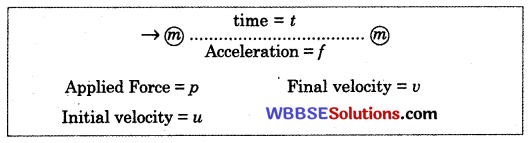So, the change of momentum after time t = (mv-mu).
Rate of change of momentum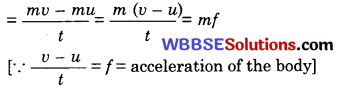Now, according to Newton’s second law of motion, rate of change of momentumNow, according to Newton’s second law of motion, rate of change of momentum is directly proportional to the applied external force.

∴ p α m f
or, p = k m f

[k is a constant of proportionality]
If we now choose the unit of the force in such a way that unit force is that force which when acting on a body of a unit mass produces unit acceleration on it, then we have p = 1, m = 1, f = 1, i.e. k = 1

∴ p = m f or Force = mass × accelerationDimensions of force :
F = [M’ L’ T2]
The force is a vector quantity.

Types of units of force :

• Absolute units
• Gravitational units

Absolute units : These units are called absolute as their values are independent of the place where the measurements are made.

CGS unit Dyne: It is the force which when acting on a body of mass one gram produces in it an acceleration of one centimetre per second every second.
(b) SI unit : Newton: It is a force which when acting on a body of mass one kilogram produces in it an acceleration of one metre per second every second.

Relation between dyne and Newton :

1 Newton = 1 kg × 1 ms2
= 1000 g × 100 cms2
= 105 g.cms2
∴ 1 Newton = 105 dyneGravitational units of force : The unit of force expressed in terms of gravity is known as gravitational unit.

CGS system : gram-weight (g-wt) : The force with which the earth attracts a body of mass one gram towards its centre is called one gram-weight.

SI system : kilogram-weight (kg-wt) : The force with which the earth attracts a body of mass one kilogram towards its centre is called one kilogramweight.
1 g – wt = 981 dyne
1 kg – wt = 9.81 Newton

Impulse : The total effect of a force is called impulse and is measured by the product of the force and the time for which the force acts on the body.

Impulsive force : Impulsive force is a force of very large magnitude but of extremely short duration, which acting on a body produces a finite change of its momentum, displacement of the body during the action of the force being negligible.

The effect of the force is measured by its impulse.
Example of impulsive force : A player lowers his hands while catching a cricket ball. In doing so he increases the time of catch to reduce the momentum of the ball to zero. We know,
Impulse = force × time = change in linear momentum

So, to produce a particular change in linear momentum, if one takes longer time, then the force required would be less. Thus in catching a cricket ball, the player increases the time of catch by lowering his hands and thereby decreases the force and smaller reaction on the hands.
Newton’s third law of motion : To every action there is an equal and opposite reaction.Newton’s third law of motion gives :

1. From Newton’s third law we find that forces always occur in pairs, i.e. a single isolated force is not possible.
2. Action and reaction [Action means the force exerted by a body on another body and reaction means the force exerted by the second body on the first] can never cancel each other, as they occur on different bodies.
3. Each force produces its own effect.
4. Newton’s third law is applicable to bodies both at rest or in motion.
5. The action and reaction forces may appear due to actual contact of the two bodies or even from a distance.
6. Newton’s third law is applicable to all types of forces e.g., gravitational, electric or magnetic etc.

Examples of action and reaction forces : If action and reaction act on the same system of particles, the action and reaction forces balance each other. So there is no displacement of the system of particle.

A chair cannot be raised sitting on it. Because in this case, action and reaction act on the same system.
Firing from a gun : When a gun is fired, the shot moves forward (action) and the gun recoils back (reaction).

Newton’s second law is the real law of motion : Both Newton’s first and third laws of motion are found to be followed from Newton’s second law of motion. So Newton’s second law can be said to be the real law of motion.

The principle of conservation of linear momentum : In an isolated system of bodies, i.e. when no external force acts on the system, the vector sum of the linear momenta of all the bodies of the system is conserved and is not affected due to their mutual action and reaction.

Characteristics of the principle of conservation of linear momentum :

1. The principle of conservation of linear momentum is universal, i.e. it is applicable to both the microscopic and macroscopic system.
2. The principle of conservation of linear momentum is independent of the frame of reference.
3. The principle of conservation of linear momentum is more fundamental than Newton’s laws of motion from which it is derived.Application of the principle of conservation of linear momentum :

A man jumps to the shore from a boat: When a man jumps to the shore from a boat, the boat is pushed away from the shore. This is because the momentum of the boat is equal and opposite to that of the man in accordance with the principle of conservation of linear momentum.

When a gas is released from a gas-filled balloon, it moves in direction opposite to that of the gas. Here also following the principle of conservation of momentum, the momentum with which the gas is coming out would impart an equal and opposite momentum to the balloon and hence the balloon would move in the opposite direction.

Motion of a rocket is based on the principle of conservation of momentum. When the fuel is burnt inside a rocket the gas produced comes out through a nozzle at the bottom of the rocket at high speed. To equalise this momentum of the issuing gas, the rocket moves upward.